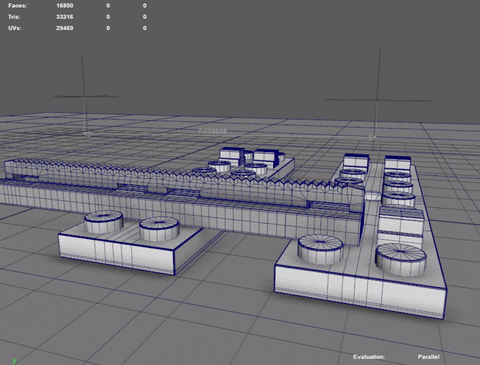# Pymel/Cmds, select a vertex, edge or lattice point by index number

#1

I am doing a little bit of modeling with python, but yet have to see once how to select components like uvs, vertices, etc. How do you access those arrays.

I seen tons about selection filters sm=31, selectType… but never in the elegant exemples do you ever see an actual selection by index number.

In this script, after creating a lattice, I would like to apply this selection:
select -cl ;
select -r ffd1Lattice.pt[0:1] ffd1Lattice.pt[0:1] ;

the lattice points i want to move.
thanks guys!

import pymel.core as pmc

firstBlockLenght = 1.0637
sectionLenght = 0.2602
midSectionLenght = 2.7884

railLenght = 11.000

spans = int((railLenght - ((firstBlockLenght * 2)+ midSectionLenght))/(sectionLenght 2))
differenceLenght = railLenght -((firstBlockLenght * 2) + midSectionLenght)-(spans
(sectionLenght*2))
upperDiffLenght = (differenceLenght - (sectionLenght 2))-1
print(differenceLenght)

railPieces = list()
for x in range(spans):
mike = pmc.duplicate(“section”, name = “section_” + str(x))
pmc.move((firstBlockLenght + (x*sectionLenght)),0,0,mike,r=True)
railPieces.append(mike)

pmc.select(railPieces)
pmc.polyUnite(muv=True,op=True,ch=False)
pmc.lattice(dv=(2,2,2), oc=True)
pmc.selectType(lp=True)
if(differenceLenght !=0):
if(upperDiffLenght < differenceLenght):
pass
(part where i move to lattice points to fit a gap between pieces)#2

pmc.select(railPieces)
pmc.polyUnite(muv=True,op=True,ch=False)
pmc.lattice(dv=(2,2,2), oc=True)
selection = mel.eval(“select -r ffd1Lattice.pt[0:1] ffd1Lattice.pt[0:1]”)

if(differenceLenght !=0):
if(upperDiffLenght < differenceLenght):
pmc.move(-upperDiffLenght,0,0, selection, r=True)
else:
pmc.move(differenceLenght,0,0, selection, r=True)

perhaps something more pythonic and direct?#3

pmc.select(‘ffd1Lattice.pt’, r=True)

this worked, wow

#4

pmc.polyUnite(muv=True, op=True, ch=False, name = ‘legoRail’)
pmc.parent()
v = pmc.polyEvaluate(‘legoRail’, v=True)
vertexString = ‘legoRail.vtx[0:’+str(v - 1)+’]'
pmc.select(vertexString)
pmc.polyMergeVertex(d=0.001, am =True, ch=False)

#5

#6

railLenght = 11 <— no
railLenght = float(11) <— yes

#7

#8pretty coo! so I can get any size rails over 5 units long
takes about a second to run